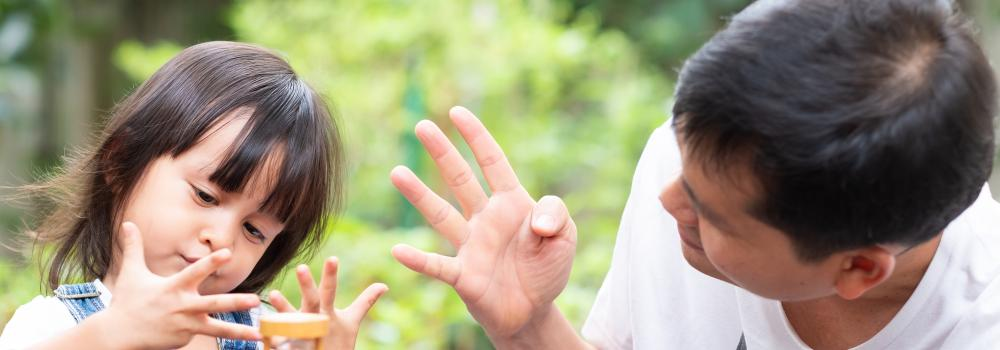# Primary/K-6: NumberNumber Sense is a vital skill that your child must learn.  While the numbers and their properties may become more sophisticated as your child grows, he/she will learn about numbers throughout his/her maths journey.

We know how fundamental learning about number is and that is why it is part of every step on our Rainbow Ladder Maths.

"Number sense develops gradually over time as a result of exploring numbers, visualising them in a variety of contexts, and relating them in ways that are not limited by traditional algorithms"
Sood and Jitendra (2007)

Here are all the topics covered in Number in our Rainbow Ladder Maths

### Red

• Counting Rhymes
• Counting
• Counting Groups
• More Than Less Than
• One More One Less Than
• Number Tracks
• Subitising
• Value of a Number
• Words for Numbers
• Number Bonds
• Estimating
• Ordinal Numbers
• Equals
• Conserving Numbers
• Subtraction
• Numerals

### Orange

• Counting with Tens
• Counting Twenty to One Hundred
• Odds and Evens
• Number Bonds to 10
• Doubles
• Using Number Tracks
• Using Number Lines
• Subtraction (Take Away)
• Subtraction (Counting Back)
• Multiplication
• Division (Sharing)
• Division (Grouping)
• Money (using 1p)
• Halves
• Quarters
• Probability

### Yellow

• Counting (10 to 20)
• Counting from any Number
• Counting in Steps
• Number Bonds (Subtraction)
• Subtraction (Counting On)
• Subtraction (Find the Difference)
• Subtraction (Using Pictures)
• Multiplication (Vocabulary)
• Multiplication Tables
• Division (Sharing)
• Division (Grouping)
• Grouping into 10s
• Counting to 20 with Bundles
• Tens and Ones
• Recording Subtraction
• Money (One to One Correspondence)
• Probability
• Empty Box Calculations
• Robots

### Green

• Counting in Steps
• Number Bonds to 20
• Subtraction using Images
• Subtraction using a Number Line
• Division with Remainders
• Halves and Quarters
• Fractions Smaller than Half
• Fractions of a Number
• Fractions and Division
• Percentages
• Hundreds, Tens and Units
• Recording Place Value
• Bar Model Maths
• Recording Multiplication
• Informal Recording of Division
• One to Many Correspondence
• Introducing 20p and 50p
• Probability

### Blue

• Counting in Steps of 25 and 50
• Subtraction (Using Numbers)
• Multiplication and Division (Arrays)
• Parts of a Fraction
• Fractions of a Number
• Percentages
• Beyond the Decimal point
• Zero as a Placeholder
• Partitioning and Re-combining Numbers
• Naming Columns
• Using Metres for Place Value
• Rounding to the Nearest 10 and 100
• Informal Recording of Calculations
• Column Recording of Addition and Subtraction
• Recording Calculations using Bars
• Swapping Coins
• Probability
• Function Machines
• Making a Function Machine
• Function Games
• Making a function Game

### Purple

• Factors and Multiples
• Negative Integers
• Special Types of Numbers
• The Grid Method
• Divisibility
• Multiplication Word Problems
• Percentages 25% and 50%
• Comparing Fractions
• Writing Amounts Using Money
• Using a Place Value Grid
• Subtraction with Decomposition
• Division with Remainders
• Investigating Algebra
• Swapping Coins
• Probability
• Probability Scale

### Lilac

• Linking Fractions, Decimals and Percentages
• Percentages Beyond 100
• Multiplying and Dividing by 10 and 100
• Decimal Numbers
• Using the Place Value Grid
• Reading and Writing Large Numbers
• Formal Recording Addition and Subtraction
• Long Multiplication
• Long Division
• Napier’s Bones
• Budgeting
• Investigating Algebra
• Roman Numerals

### Areas of study

This site uses cookies. You can opt out of receiving optional cookies at any time.